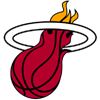DraftKings Eastern Conference Odds
+140
1.4 to 1Nets
34.4% implied probability

+320
3.2 to 1Bucks
19.6% implied probability

+650
6.5 to 176ers
11.0% implied probability

+700
7 to 1Celtics
10.3% implied probability

+700
7 to 1Heat
10.3% implied probability

+1600
16 to 1Pacers
4.8% implied probability

+2000
20 to 1Raptors
3.9% implied probability

+5000
50 to 1Hawks
1.6% implied probability

+6000
60 to 1Magic
1.4% implied probability

+10000
100 to 1Wizards
0.8% implied probability

+15000
150 to 1Hornets
0.5% implied probability

+25000
250 to 1Bulls
0.3% implied probability

+25000
250 to 1Cavaliers
0.3% implied probability

+25000
250 to 1Pistons
0.3% implied probability

+25000
250 to 1Knicks
0.3% implied probability